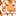# QlikView App Dev

Discussion Board for collaboration related to QlikView App Development.

cancel
Showing results for
Did you mean:Creator

## Nested IF with AND operator in Qlik Sense

Hi everyone,

i have a problem when i try to create a new calculated field by using a Nested IF.

For example I have a Field Age and I want create a new Field (Color) with 'A' if Age > 10 and < 20, 'B' if Age >=20 and < 40 and so on...

I try to create this function but Qlik show me this error: missing right parenthesis:

 `if``(``Age` `> 10 and ``Age` `< 20,A,`

 `7` ` ``if``(``Age` `>= 20 and ``Age` `< 40,B,))`

Thanks,

Livio

1 Solution

Accepted SolutionsMVP

You may miss understanding the IF condition

If([City Code]> 10 and [City Code] <20, 'A', If([City Code] >= 20 and [City Code] < 40, 'B', 'C'))

Explanation - If [City Code] arbitrary between 10 and 20 then it returns only A and [City Code] arbitrary between 20 and 40 then it returns only B. That means, If [City Code] < 10 and [City Code] >40 it returns C only. The same way your functionality worksBefore develop something, think If placed (The Right information | To the right people | At the Right time | In the Right place | With the Right context)
36 RepliesPartner - Master III

Share full expression..MVP

Try to create like below

If(Age>100 and Age<20, 'A', If(Age>=20 and Age<40, 'B'))

Before develop something, think If placed (The Right information | To the right people | At the Right time | In the Right place | With the Right context)MVP

Remove last comma or put a else value, like:

`.....if``(``Age` `>= 20 and ``Age` `< 40,B))`

`OR`

`if(Age >= 20 and Age < 40,B, C))`Creator

HI,

You seem to have forgotten the default value.

 `if``(``Age` `> 10 and ``Age` `< 20,'A',`

 `7` `if``(``Age` `>= 20 and ``Age` `< 40,'B',???))`

Filling up the ??? will help you. else remove the comma after B.

Hope this helpsCreator
Author

Hi,

i have try to create another calculated field like this:

If(City Code> 100 and City Code <20, 'A', If(City Code >= 20 and City Code < 40, 'B'))

But Qlik show me the same errorCreator
Author

If(City Code> 100 and City Code <20, 'A', If(City Code >= 20 and City Code < 40, 'B'))Master III

Just for reference ...

if([Sale>=10 and Sale <=20   ,Green(),

if(Sale >20 ,Blue(),

if(Sale >0 and Sale <10 ,Black(),

rgb(255,0,0))) )Creator
Author

No, qlik show me the same error....

if(City Code>=10 and City Code <=20   ,Green(),

if(City Code >20 ,Blue(),

if(City Code >0 and City Code <10 ,Black(),

rgb(255,0,0))) )Creator
Author

No, nothing. Qlik show me the same error